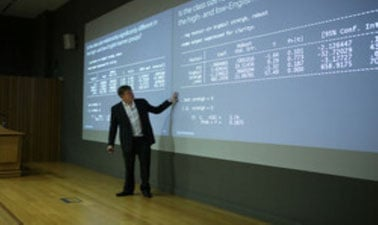# The London School of Economics and Political Science: Statistics 1 Part 1: Introductory statistics, probability and estimation

4.3 stars
11 ratings

The first in a series of four courses which help you to master statistics fundamentals and build your quantitative skillset for progression in high-growth careers, or to use as step towards further study at undergraduate level.5 weeks
12–15 hours per week
Self-paced
Free

Statistics 1 Part 1 is a self-paced course from LSE which aims to introduce you to and develop your understanding of essential statistical concepts, methods and techniques, emphasising the applications of these methods. This course can be taken alone or as part of the LSE MicroBachelors program in Statistics Fundamentals or the LSE MicroBachelors program in Mathematics and Statistics Fundamentals.

Part 1, Introductory Statistics, Probability and Estimation, covers the following topics:

● Mathematical revision and the nature of statistics

● Data visualisation and descriptive statistics

● Probability theory

● The normal distribution and ideas of sampling

● Point and interval estimation

Statistics 1 Part 1 forms part of a series of courses which focuses on the application of statistical methods in management, economics and the social sciences. During this course, you will focus on the interpretation of tables and results, and how to approach statistical problems effectively.

### At a glance

• Institution: LSE
• Subject: Data Analysis & Statistics
• Level: Intermediate
• Prerequisites:

Statistics 1 Part 1 assumes no prior knowledge of statistics. Essential statistical concepts are introduced and are further built on in Statistics 1 Part 2 (and also in Statistics 2, Parts 1 and 2). It is strongly recommeded to study the LSE statistics courses in order (progressing to Statistics 1, Part 2) given the cumulative nature of the subject matter.

• Language: English
• Video Transcript: English
• Associated programs:
• Associated skills: Management, Normal Distribution, Social Sciences, Statistical Methods, Sampling (Statistics), Economics, Probability Theories, Probability, Basic Math, Statistics, Data Visualization

# What you'll learn

Skip What you'll learn

By the end of this course, you will:

• be familiar with some key ideas of statistics that are accessible to a student with a moderate mathematical competence

• be able to routinely apply a variety of methods for explaining, summarising and presenting data and interpreting results clearly using appropriate diagrams, titles and labels

• have a grounding in probability theory

# Syllabus

Skip Syllabus

● Mathematical revision and the nature of statistics

● Data visualisation and descriptive statistics

● Probability theory

● The normal distribution and ideas of sampling

● Point and interval estimation